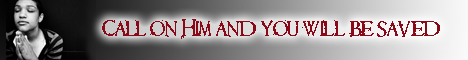Note:  Do not rely on this information. It is very old.

# Polygon

Polygon is any figure whose sides are straight lines; when these sides are all equal and include equal angles, the polygon is said to be regular. All regular polygons can be circumscribed to and inscribed in a circle, but the problem is not always easy of solution. Euclid devised methods for performing the operation with polygons of 3, 4, or 5 sides; and hence the method can be applied to cases where the number of sides is 3, 4, or 5 multiplied by any power of 2. Gauss gave solutions for polygons of a more complicated type. All polygons of an even number of sides, if circumscribed about a circle, have the sums of the odd and even sides equal; and, if inscribed in a circle, the sums of the odd and even angles are equal. The sum of the angles of any polygon is equal to four less than twice as many right angles as the figure has sides.